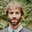Question-and-Answer Resource for the Building Energy Modeling Community
Get started with the Help page

# Modelica control of constant input (Type Real)I have a real constant serving as the source for a linear valve in Modelica. I would like to control this input.

I tried redefining the parameter k=k: "parameter Real k = 0.5;" --> works fine, gives a result!

Then if I redefine as "parameter Real k;" and try defining "k = 0.5;" under equations, I get the error that there are one too many equations. Okay...kind of makes sense as I did add one but I only defined k once.

My goal was to define k in equations so that I could set up a controller to stabilize flow. Should I be able to do this? If not, is there another method I should be trying?

Thanks!

edit retag close merge delete

Sort by » oldest newest most voted

Update. It turns out I can get rid of the real constant icon and redefine this term as something like valve.opening = 0.5 in the equation section.

more